Saturday, 26 December 2015

Fiscal Policy in Neo-Fisherite Versions of New Keynesian Models

This post is intended to enable me to explain in a bit more detail something I have been describing in an exchange with Stephen Williamson on his blog.

The context is how fiscal policy might impact on the results of models of interest rate changes, particularly in the impulse response on output.  I have in mind here a model based on John Cochrane's, which I've looked at before.  The two main equations are a New Keynesian IS curve and Phillips curve.  There are also assumed to be lump sum taxes and single period government bonds, so there needs to be a government budget constraint which determines the evolution of the stock of bonds.  The three equations in y (output), π (inflation) and b (the real end period value of the stock of bonds) are:

(1)          yt = E[yt+1] . ( β . it+1 / E[πt+1] )

(2)          πt = E[πt+1]β . ytκ

(3)          bt = bt-1 . it / πt - τt

where i is the interest rate and τ is the level of real lump sum taxes.

Here, monetary policy is an interest rate peg with i as the policy tool - we have no central bank reaction function.  We want to consider the impact of a 1% cut in this rate, announced five period in advance.  In the first instance, we will assumes that fiscal policy involves keeping τ constant.

The results of this are shown below (showing deviations from starting values).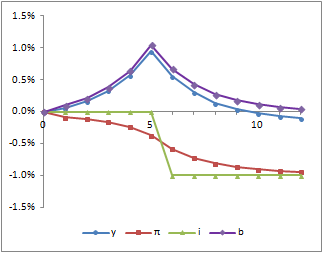Inflation starts to decline immediately and eventually reflects the drop in nominal interest rates.  This means that, prior to the actual rate cut, real interest rates are higher, which implies that output rises.  Once actual nominal rates are cut, real rates are lower and output declines again.

This change results in a temporary increase in the real stock of bonds.  This happens because real rates have risen and real rates are a key factor in determining how the stock of bonds changes over time.

So I want to consider an alternative fiscal policy rule; one where the level of taxes, τt, is adjusted to prevent bt ever rising above b0.  We therefore need a fourth equation which is:

(4)          τt = GREATER OF { τ0 } OR { bt-1 . it / πt - b0 }

Adding in this equation gives us the results shown below (bt = b0 for all t, so I have not included it in the chart).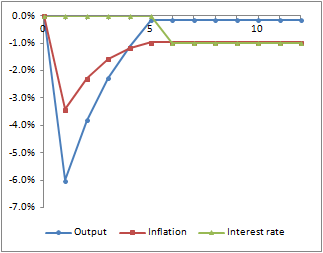Under this fiscal policy rule, both output and inflation drop sharply on the announcement, and then both rise until the actual rate change takes place.  These paths clearly satisfy equations (1) and (2) for every period where there is no surprise.  Output is rising when inflation is lower than nominal interest rates; inflation is rising when output is below its equilibrium level.  Equations (3) and (4) selects this equilibrium path from multiple others that would also satisfy (1) and (2) (although I cannot be sure that there are no other alternative paths that would satisfy all four equations).

Note on parameters.  I have used Cochrane's values of β = 0.97 and κ = 0.2, (as well as assuming a τ0 equal to 20% of y0).  These parameters suggest time periods in the order of a year, so we are talking about changes announced five years in advance.  If we adjust the parameters to reflect shorter periods (like a month), then announcing five periods in advance has a more limited impact (although still the same pattern).

Friday, 18 December 2015

Money as a Good and Money as a Record

In a recent post, Nick Rowe has been playing around with how to think about money when measured money can be both posive and negative.

It is worth distinguishing two models of money, which on the face of it are very different.

In the first model, money is a type of good.  Monetary exchange means that every purchase involves the buyer delivering the agreed amount of money to the seller in return for goods and services.  It's useful to imagine this as a physical delivery - the buyer hands over some gold coins or paper notes to the seller.

In the second model, monetary exchange takes place through a series of accounts kept by a central registry (the Bank).  Each agent holds an account, which is nothing more than a record of a balance at the close of each day.  When a purchase takes place, the buyer requests the Bank to debit the purchase price from his own account and credit it to that of the seller.

In each case there is a limit on how much people can spend.  In the first model, this is a physical limit.  The buyer cannot hand over more paper notes than he actually has - you can't have negative notes.

In the second model, there is no physical limit.  If the buyer spends more than he holds, his balance simply becomes negative.  However, the Bank will want to impose some limits.  The more an account goes negative, the greater the risk to the Bank if the accountholder fails to get it positive again.  So the Bank will decline payment requests which will make accounts more negative than it wants.

So in the first model, the limit is necessarily zero.  In the second model, it may be any non-positive number and will depend on the Bank's view on credit risk.

This question of limits is the essential difference between the two models, rather than any question of whether money is in bearer or registered form.  Of course, the form money takes may dictate the limit - you can't have negative bearer notes.  But it's the limits that matter.

This allows us to see that the first model is simply a special case of the second model.  In the second model, the limit need only be non-positive.  In the first model, it must be zero.

Negative account balances in the second model are debts of the accountholder.  We can have debts in the first model as well, where the Bank (or any other agent) lends notes to someone.  However, there is a clear distinction here between debts and money.  Although the paper notes may constitute debts of the Bank, as far as the non-banking sector is concerned debts and money are quite different things.

This makes it easy to isolate a particular thing - in this case the collection of paper notes - and identify it as money or the medium of exchange.  Helpfully, such a thing can be captured in a single aggregate measure.  So, it's often easier to think through monetary mechanics using the first model, and often this is OK.

However, in the general case the distinction between medium of exchange and debt becomes more blurred.  We can no longer think of money as a simple aggregate; we need to start to think about the general liquidity position.  This manifests itself in the way counterparties to monetary contracts - both banks and non-banks - agree the terms under which those contracts may be settled on demand.

The second model is a closer reflection of the real world.  We can learn useful things from thinking about models where money is a good with a strictly positive value.  But we should not be misled into believing that everything we see in those models will translate neatly to the real world.

Saturday, 12 December 2015

A Portfolio Balance Model of Exchange Rates

In my last post, I explained how I like to think about the relationship between exchange rates and international balances.  The key point was that current flows do not matter much in themselves.  What matters is the build up of balances, in particular where those involve entities having to take positions in their non-functional currency.

I like models, so I'm using this post to set out a little model of this process.  This is based on the models of Godley and Lavoie.  The most notable departure is that I am setting the expected exchange rate equal to the actual outcome.  I'm doing this because the results of this type of model can depend heavily on how exchange rate expectations are formed.  To fully understand this, we need to see how the models behave when we eliminate any systematic expectation error.

Unfortunately, open economy models with floating exchange rates generally require many more equations than closed economy models.  To keep this manageable enough to contain in a blog post, I have made the model as simple as I can whilst retaining enough to show the key dynamics.  The main point here is that I have assumed a "small" economy, so that I can take what goes on in the rest of the world as exogenous.   As usual, I have relegated the equation listing to the end.

There are three sectors: a public sector, a domestic private sector and the rest of the world.  There are two financial assets - domestic government bonds and foreign government bonds.  Both are held by both domestic and foreign investors.  This is shown in the balance sheet matrix below.  As I have consolidated foreign investors and foreign issuers into one, foreign holdings of foreign bonds do not appear.  Foreign bonds are recorded at their foreign currency value and divided by the exchange rate so the matrix is all in the domestic currency.

 Private Sector Government Rest of World Domestic bonds Bd -B Bw Foreign bonds Fd / e - Fd / e Total V -B NFI

The net wealth of each sector is determined by historical flows to date (subject to valutaion at the prevailing exchange rate).  The exchange rate must then adjust to ensure that investors wish to hold domestic and foreign bonds in the proportions in which they are in issue.

There are two portfolio decisions to be made here.  First, the domestic private sector has to decide how to allocate its financial wealth between domestic and foreign bonds.  This is assumed to be a function of the domestic interest rate (r) and the expected return on foreign bonds (rrf).  This latter return needs to take into account expected exchange rate movements.

Fd / e = f1 ( V, r, rrf )

The amount of domestic bonds that foreign investors wish to hold is also assumed to depend on rates.  Here the rates are the domestic rate adjusted for expected exchange rate movements and the foreign rate.  The function also depends on total overseas financial wealth (Vf), converted to its domestic equivalent value.  As we are assuming a small economy, Vf is treated as exogenous.

Bf = f2 ( Vf / e, rrd , rf )

The other behavioural equations required in the model are those describing expenditure - total private expenditure, exports and imports - and those describing how domestic prices adjust.  I have used similar expenditure functions to those that appear in G&L with prices determined by a Phillips curve type relationship based on adaptive expectations.  (It would not be too difficult to adapt this to include some more micro-founded behavioural assumptions and a forward looking Phillips curve.)  I have also assumed there is no intermediate production (imports are not used in production of exports).

The accounting structure of the model is best captured by the flow of funds matrix.  (This includes a production account so that all rows sum to zero, as well as all columns.)

 Private Sector Government Rest of World Production Factor income Y - Y Taxes - T T Domestic interest r . Bd - r . B r . Bw Foreign interest rf. Fd / e - rf . Fd / e Domestic consumption - d . p d . p Government spending - g . p g . p Exports - x . p x . p Imports - m . pf / e m .  pf / e Change in domestic bonds - Δ Bd Δ B - Δ Bw Change in foreign bonds - Δ Fd / e Δ Fd / e Total 0 0 0 0

There are lots of things we can explore with this model, including many of those typically explored within Mundell-Fleming style models.  As an example, I have looked at what happens when there is a sudden unexpected change in the preferences of domestic investors towards foreign assets.  The charts below show some of the results.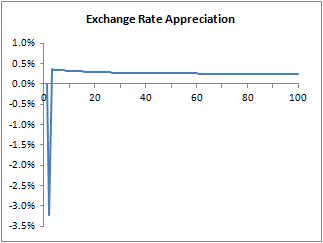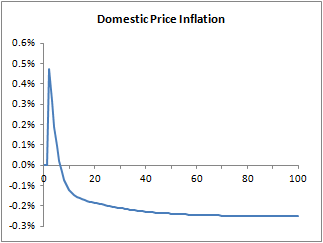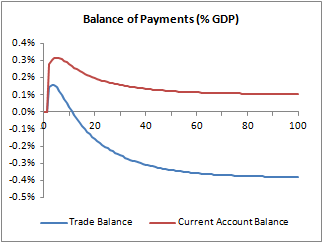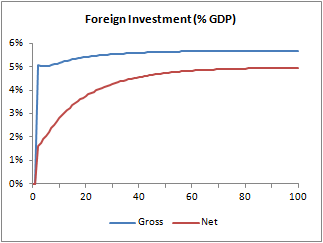The immediate impact is a sharp drop in the exchange rate.  Domestic investors purchase more foreign bonds (reflected in the jump in gross overseas investment), but in the short run the current account flows are insufficient to finance this.  The counterpart must therefore be purchase by foreign investors of the domestic bonds that domestic investors are selling.  The exchange rate falls until foreign investors expect sufficient future currency gains to make them want the additional domestic bonds.  The initial impact on net foreign investment is principally due to the upwards revaluation of overseas investment due to the exchange rate movement.

The drop in the exchange rate gives a boost to net exports, which together with rising import prices gives a brief increase in domestic inflation.  However, having initially fallen, the exchange rate now rises (which delivers the higher returns expected by foreign investors).  The combined effect erodes the price advantage in foreign trade and the initial increase in the trade balance is soon reversed.  However, by then the net foreign investment position has improved enough that net interest income from abroad outweighs the negative trade balance.

In the long-term steady-state position, lower domestic inflation is matched by currency appreciation, so the real exchange rate is constant.  The higher current account balance is offset by the impact of currency appreciation on the capital losses to domestic investors on foreign bondholdings.

There are many interesting dynamics that are brought out by this model.  I might look at some more in subsequent posts.

Equation Listing

Real output is the sum of domestic private expenditure, government expenditure and exports.

(1)          y =  d + g + x

The real value of domestic private expenditure is total nominal private expenditure less nominal imports, divided by the price of domestic goods.

(2)          d = ( C - m . pf / e ) / p

Total private expenditure is based on disposable income and the stock of bonds held:

(3)          C = α1 . YD  + α2 . ( Bd-1 + Fd-1 / e )

where disposable income is based on current flows, but excludes capital gains and losses (part of the reason for doing this is to avoid blips in disposable income due to revaluations in foreign bonds when the exchange rate jumps.)

(4)          YD = y . p - T + r-1 . BD-1 + rf . Fd-1 / e

Taxes are levied as a proportion (τ) of production income and nominal interest income.

(5)          T = τ .  ( y . p + r-1 . Bd-1 + rf . Fd-1 / e )

The ratio of real imports to real domestic private expenditure is based on the terms of trade.

(6)          m = d . µ . ( e . p / pf )σ1

Real exports are based on the terms of trade.

(7)          x = x0 . ( e . p / pf )σ2

The definition of disposable income implies that private wealth evolves according to the following accounting identity:

(8)          V = YD - C + Bd-1 + Fd-1 / e

The domestic private sector allocates a portion of its wealth to foreign bonds based on relative expected returns.

(9)          Fd = e . λ1 . V. ( rrf / r )κ1

Foreign holdings of domestic bonds is based on the relative rates of return and the stock of the rest of the world's savings (converted into the domestic currency equivalent).

(10)        Bw = λ2 . Vf / e . ( rrd / rf )κ2

The stock of domestic bonds evolves in line with the government budget constraint.

(11)        B = B-1 . ( 1 +r-1 ) + g . p - T

The balance of wealth is made up of domestic bonds

(12)        Bd = V - Fd / e

The balance of domestic bonds are held by overseas investors.

(13)        Bw = B - Bd

(We already have an equation for Bw.  For the purposes of solving the model, this equation (10) is re-arranged to define the exchange rate)

The expected rate of return (expressed in foreign currency terms) on domestic bonds depends on the nominal domestic interest rate and expected exchange rate movements.

(14)        rrd = ( 1 + rd ) . E[ e+1 ] / e - 1

(15)        rrf = ( 1 + rf ) . e / E[ e+1 ] - 1

Price setting here is based on inflation expectations and a measure of the output gap.

(16)        p = p-1 . E[π] . ( y / y* )ψ

(17)        E[π] = E[π]-1 + ε . ( π-1 - E[π]-1 )

Where inflation is taken as the change in the price of domestic output (expressed as 1 plus the change).

(18)        π = p / p-1

Variables

The variables are listed below.  Uppercase variables denote nominal values.

 B Domestic bonds Bd Domestic bonds held by domestic investors Bw Domestic bonds held by overseas investors D Domestic expenditure by the domestic private sector e Exchange rate (units of foreign currency per unit of domestic currency) Fd Overseas bonds held by domestic investors (in foreign currency terms) g Real government expenditure m Real imports p Price of domestic output pf Price of foreign output r Interest rate on domestic bonds rf Interest rate on foreign bonds rrd Expected return to foreign investors on domestic bonds rrf Expected return to domestic investors on foreign bonds T Taxes V Net financial wealth of domestic private sector Vf Financial wealth of rest of world (in foreign currency terms) X Real exports x0 Base level of real exports y Real output YD Disposable income of domestic private sector π Inflation of domestic output prices

Policy variables are the level of government expenditure, the tax rate (τ) and the domestic interest rate.  Foreign variables (Vf, x0 and rf) are taken as exogenous in accordance with the "small" economy assumption.